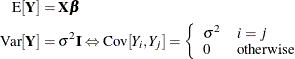### Linear Model Theory

Subsections:

This section presents some basic statistical concepts and results for the linear model with homoscedastic, uncorrelated errors in which the parameters are estimated by ordinary least squares. The model can be written as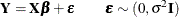where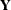is an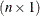vector andis an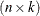matrix of known constants. The model equation implies the following expected values: